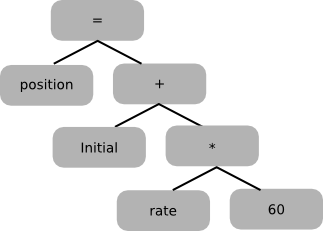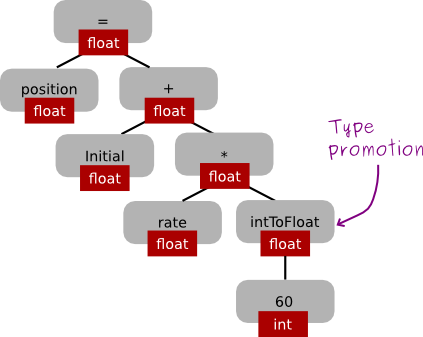Contents

What is a compiler?

• A recognizer (of some source language L).
• A translator (of programs written in L into programs written in some object or target language L').
Here's a simple pictorial view:

A compiler is itself a program, written in some host language. (In cs536, students will implement a compiler for a simple source language using Java as the host language.)

A compiler operates in phases; each phase translates the source program from one representation to another. Different compilers may include different phases, and/or may order them somewhat differently. A typical organization is shown below.

Below, we look at each phase of the compiler.

The scanner is called by the parser; here's how it works:

• The scanner reads characters from the source program.
• The scanner groups the characters into lexemes (sequences of characters that "go together").
• Each lexeme corresponds to a token; the scanner returns the next token (plus maybe some additional information) to the parser.
• The scanner may also discover lexical errors (e.g., erroneous characters).
The definitions of what is a lexeme, token, or bad character all depend on the source language.

### Example

Here are some Java lexemes and the corresponding tokens:

```lexeme:                 ;           =        index    tmp    37        102

corresponding token:  SEMI-COLON   ASSIGN    IDENT  IDENT  INT-LIT  INT-LIT
```

Note that multiple lexemes can correspond to the same token (e.g., there are many identifiers).

Given the source code:

```position  =  initial +  rate   *    60 ;
```
a Java scanner would return the following sequence of tokens:
```IDENT  ASSIGN IDENT PLUS IDENT TIMES INT-LIT SEMI-COLON
```
Erroneous characters for Java source include # and control-a.

The Parser

• Groups tokens into "grammatical phrases", discovering the underlying structure of the source program.
• Finds syntax errors. For example, in Java the source code
position = * 5 ;
corresponds to the sequence of tokens:
IDENT ASSIGN TIMES INT-LIT SEMI-COLON
All are legal tokens, but that sequence of tokens is erroneous.
• Might find some "static semantic" errors, e.g., a use of an undeclared variable, or variables that are multiply declared.
• Might generate code, or build some intermediate representation of the program such as an abstract-syntax tree.

### Example

source code:   `position = initial + rate * 60 ;`

Abstract syntax tree:Notes:

• The interior nodes of the tree are operators.
• A node's children are its operands.
• Each subtree forms a "logical unit", e.g., the subtree with * at its root shows that because multiplication has higher precedence than addition, this operation must be performed as a unit (not initial+rate).

The semantic analyzer checks for (more) "static semantic" errors, e.g., type errors. It may also annotate and/or change the abstract syntax tree (e.g., it might annotate each node that represents an expression with its type). Example:

Abstract syntax tree before semantic analysis:Abstract syntax tree after semantic analysis:The intermediate code generator translates from abstract-syntax tree to intermediate code. One possibility is 3-address code (code in which each instruction involves at most 3 operands). Below is an example of 3-address code for the abstract-syntax tree shown above. Note that in this example, the second and third instructions each have exactly three operands (the location where the result of the operation is stored, and two operators); the first and fourth instructions have just two operands ("temp1" and "60" for instruction 1, and "position" and "temp3" for instruction 4).

```		temp1 = inttofloat(60)
temp2 = rate * temp1
temp3 = initial + temp2
position = temp3
```

The optimizer tries to improve code generated by the intermediate code generator. The goal is usually to make code run faster, but the optimizer may also try to make the code smaller. In the example above, an optimizer might first discover that the conversion of the integer 60 to a floating-point number can be done at compile time instead of at run time. Then it might discover that there is no need for "temp1" or "temp3". Here's the optimized code:

```		temp2 = rate * 60.0
position = initial + temp2
```

The code generator generates object code from (optimized) intermediate code. For example, the following code might be generated for our running example:

```                .data
c1:
.float  60.0
.text
l.s     \$f0,rate
mul.s   \$f0,c1
l.s     \$f2,initial